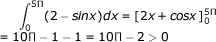# Calculus - Calculus MCQ

1:

IfA. 2x2 B. √x C. 0 D. 1 Answer Report Discuss Option: A Explanation :Click on Discuss to view users comments. Sagnik Bhawal said: (1:56pm on Wednesday 5th August 2015) while integrating how are the upper and lower limits getting changed? coz the integration doesn't result a negative sign. In absence of a negative sign how are the limits getting interchanged? i mean the answer should have been -2x^2 instead of 2x^2 ravi said: (5:48am on Saturday 5th December 2015) it should be -2x^2 Waqar said: (1:57pm on Thursday 4th May 2017) Dear, Its Answer Should be -2x square not 2x square. how can you exchange limits? Write your comments here:
2:

The function f(x) = x3 - 6x2 + 9x + 25 has

 A. a maxima at x= 1 and a minima at x = 3 B. a maxima at x = 3 and a minima at x = 1 C. no maxima, but a minima at x = 1 D. a maxima at x = 1, but no minima Answer Report Discuss Option: A Explanation : f '(x) = 3(x2-4x+3)    f ''(x)=6(x)-12 at  x=1,  f "(1)   at  x=3, f ''(3) = +ve Click on Discuss to view users comments. abc said: (9:22am on Friday 5th April 2013) how x=1 and x=3 arrive?? Akem said: (1:02pm on Tuesday 8th September 2015) i taught maxima at x=3 because is positive and minima at x = 1 because it is negative Jay Vyas said: (4:14pm on Friday 27th November 2015) at X = 1 We got minimum value and at X= 3 we got maximum valueSo in my opinion option B is right Samrah said: (2:38am on Wednesday 13th July 2016) Take 1st derivative of the function and put it equal to 0 to find the value of "x".Now take 2nd derivative and put the 2 values of X previously found in equation to get a result respectively. If the final answer is ve so it is a Minima. If the answer is -ve it is a maxima. Write your comments here:
3:

The value of a =is

 A. >0 B. 2 C. 0 - 1 + 100 - 10 + 1 D. undefined Answer Report Discuss Option: A Explanation :Click on Discuss to view users comments. kiran said: (2:23am on Friday 2nd December 2016) how cos5p=-1 Write your comments here:
4:

The interval in which the Lagrange's theorem is applicable for the function f(x) = 1/x is

 A. [-3, 3] B. [-2, 2] C. [2, 3] D. [-1, 1] Answer Report Discuss Option: C Explanation : Since f(x) = (1/x) is not continuous in [- 3, 3] [- 4, 2] or [- 1, 1], The point of discontinuity is '0'. Only in [2, 3] the function is continuous, and differentiable hence mean value theorem is applicable in [2, 3]. Click on Discuss to view users comments. Write your comments here:
5:

If f(x) = | x | , then for interval [-1, 1] ,f(x)

 A. satisied all the conditions of Rolle's Theorem B. satisfied all the conditions of Mean Value Theorem C. does not satisied the -conditions of Mean Value Theorem D. None of these Answer Report Discuss Option: C Explanation : Since f(x) = |x| is continuous in [-1, 1] but  it is not differentiable at x = 0 ε (-1, 1) Click on Discuss to view users comments. Write your comments here: